Next: Utilities Up: Analyzing univariate polynomials Previous: Analyzing the real roots   Contents

Analyzing the real part of the roots

For some applications it may be interesting to determine if a polynomial in a set defined by a parametric polynomial has the real part of one of its roots equal to a pre-defined value. This may be done by using the procedure

int ALIAS_Is_Root_RealPart(int Degree,
int NbParameter,
int HasInterval,
INTERVAL_VECTOR (* TheCoeff)(INTERVAL_VECTOR &),
INTERVAL_VECTOR (* TheCoeffCentered)(INTERVAL_VECTOR&,double a),
int Iteration,
INTERVAL_VECTOR &Par,
double *Root,
INTERVAL_VECTOR (* EvaluateComplex)(int,int,INTERVAL_VECTOR &),
int (* Simp)(INTERVAL_VECTOR &))
where
• Degree: the degree of the polynomial
• NbParameter: the number of parameters that appear in the coefficient of the polynomial
• HasInterval: 1 if the coefficient include intervals not defined by parameters, 0 otherwise
• TheCoeff: a procedure to calculate the coefficients of the polynomial being given range for the parameters
• TheCoeffCentered: a procedure to calculate the coefficients of the polynomial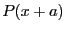• Iteration: the maximum number of box that may be used by the algorithm
• Par: the ranges for the parameters
• Root: we look for polynomial whose real part of the root is either Root or Root
• EvaluateComplex: a procedure that returns 4 intervals. The input interval vector has dimension NbParameter+1 elements, the first one being the parameters, the last one being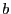. The procedure should evaluate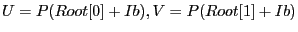and should return the real part of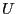, the complex part of, the real part of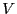and the complex part of• Simp: an optional simplification procedure that returns -1 if the polynomials in a set cannot roots with real part equal to Root or RootNext: Utilities Up: Analyzing univariate polynomials Previous: Analyzing the real roots   Contents
Jean-Pierre Merlet 2012-12-20